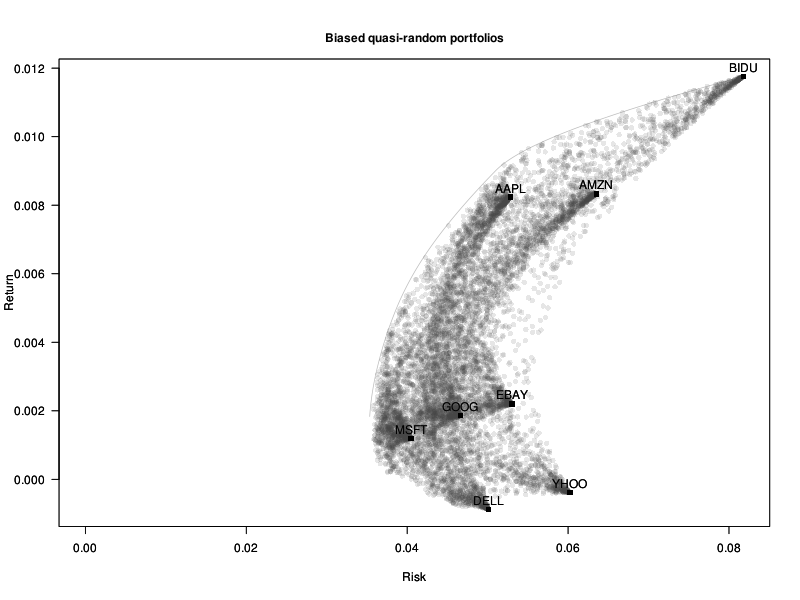# Vincent Zoonekynd's Blog

## Fri, 01 Jun 2012: Optimization

Many problems in statistics or machine learning are of the form "find the values of the parameters that minimize some measure of error". But in some cases, constraints are also imposed on the parameters: for instance, that they should sum up to 1, or that at most 10 of them should be non-zero -- this adds a combinatorial layer to the problem, which makes it much harder to solve.

In this note, I will give a guide to (some of) the optimization packages in R and explain (some of) the algorithms behind them. The solvers accessible from R have some limitations, such as the inability to deal with binary or integral constraints (in non-linear problems): we will see how to solve such problems.

When you start to use optimization software, you struggle to coax the problem into the form expected by the software (you often have to reformulate it to make it linear or quadratic, and then write it in matrix form). This is not very user-friendly. We will see that it is possible to specify optimization problems in a perfectly readable way.

```# Actual R code
x <- variable(5)
minimize( sum(abs(x)) + sum(x^2) - .2*x*x )
x >= 0
x <= 1
sum(x) == 1
x == x
r <- solve()```

Many of the examples will be taken from finance and portfolio optimization.More...

posted at: 11:45 | path: /R | permanent link to this entry